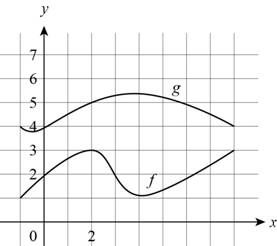# The values of functions ( f + g ) ( 2 ) , ( f − g ) ( 2 ) , ( f ⋅ g ) ( 2 ) and ( f g ) ( 2 ) from the given graph.### Precalculus: Mathematics for Calcu...

6th Edition
Stewart + 5 others
Publisher: Cengage Learning
ISBN: 9780840068071### Precalculus: Mathematics for Calcu...

6th Edition
Stewart + 5 others
Publisher: Cengage Learning
ISBN: 9780840068071

#### Solutions

Chapter 2.6, Problem 1E
To determine

## To evaluate: The values of functions (f+g)(2) , (f−g)(2) , (f⋅g)(2) and (fg)(2) from the given graph.

Expert Solution

The values of (f+g)(2) is 8, (fg)(2) is 2_ (fg)(2) is 15 and (fg)(2) is 35 .

### Explanation of Solution

Given:

The graphs of f and g is given below,Figure (1)

Graphs of function f(x) and g(x) are shown in Figure (1).

Calculation:

From the Figure (1), in the graph of y=f(x) , the value of 2 at x-axis is at 3 of y-axis, so the value of f(2) is 3.

From the Figure (1), in the graph of y=g(x) , the value of 2 at x-axis is at 5 of y-axis, so the value of g(2) is 5.

Calculate the sum of the both the functions by below formula,

(f+g)(x)=f(x)+g(x) .

Substitute 2 for x in above formula,

(f+g)(2)=f(2)+g(2) .

Substitute 3 for f(2) and 5 for g(2) in above formula,

(f+g)(2)=3+5=8

Thus, the values of (f+g)(2) is 8.

Now, from the Figure (1), in the graph of y=f(x) , the value of 2 at x-axis is at 3 of y-axis, so the value of f(2) is 3.

From the Figure (1), in the graph of y=g(x) , the value of 2 at x-axis is at 5 of y-axis, so the value of g(2) is 5.

Calculate the difference of the both the functions by below formula,

(fg)(x)=f(x)g(x) .

Substitute 2 for x in above formula,

(fg)(2)=f(2)g(2) .

Substitute 3 for f(2) and 5 for g(2) in above formula,

(fg)(2)=35=2

Thus, the value of (fg)(2) is 2_

Similarly, From the Figure (1), in the graph of y=f(x) , the value of 2 at x-axis is at 3 of y-axis, so the value of f(2) is 3.

From the Figure (1), in the graph of y=g(x) , the value of 2 at x-axis is at 5 of y-axis, so the value of g(2) is 5.

Calculate the product of two functions by below formula,

(fg)(x)=f(x)g(x) .

Substitute 2 for x in above formula,

(fg)(2)=f(2)g(2) .

Substitute 3 for f(2) and 5 for g(2) in above formula,

(fg)(2)=35=15

Thus, the value of (fg)(2) is 15.

From the Figure (1), in the graph of y=f(x) , the value of 2 at x-axis is at 3 of y-axis, so the value of f(2) is 3.

From the Figure (1), in the graph of y=g(x) , the value of 2 at x-axis is at 5 of y-axis, so the value of g(2) is 5.

Calculate the product of two functions by below formula,

(fg)(x)=f(x)g(x)

Substitute 2 for x in above formula,

(fg)(2)=f(2)g(2)

Substitute 3 for f(2) and 5 for g(2) in above formula,

(fg)(2)=35

Thus, the value of (fg)(2) is 35 .

### Have a homework question?

Subscribe to bartleby learn! Ask subject matter experts 30 homework questions each month. Plus, you’ll have access to millions of step-by-step textbook answers!